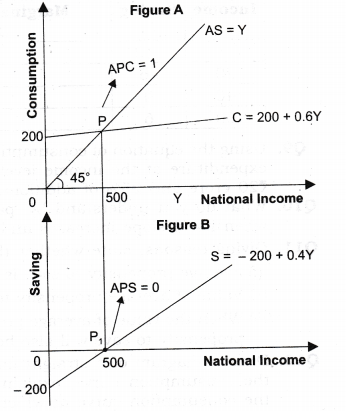# NCERT Solutions Class 12 Macro Economics Chapter-5 (Aggregate Demand and Its Related Concepts)

NCERT Solutions Class 12 Macro Economics from class 12th Students will get the answers of Chapter-5 (Aggregate Demand and Its Related Concepts) This chapter will help you to learn the basics and you should expect at least one question in your exam from this chapter.
We have given the answers of all the questions of NCERT Board Macro Economics Textbook in very easy language, which will be very easy for the students to understand and remember so that you can pass with good marks in your examination.

Class 12 Macro Economics

Chapter-5 (Aggregate Demand and Its Related Concepts).

Questions and answers given in practice

Chapter-5 (Aggregate Demand and Its Related Concepts)

NCERT TEXTBOOK QUESTIONS SOLVED

Question 1. What is marginal propensity to consume? How is it related to marginal propensity to save?[3-4 Marks]
Answer(i) The ratio of change in consumption (C) to change in income (Y) is known as marginal propensity to consume. It indicates the proportion of additional income that is being spent on consumption.
MPC=ΔCΔY
The sum of MPC and MPS is equal to one. It can be proved as under: We know: ΔY = ΔC + ΔS
Dividing both sides by AY, we get,ΔY/ΔY=ΔC/ΔY + ΔS/ΔY or 1=MPC+MPS
[ΔY/ΔY=1; ΔC/ΔY =MPC ΔS/ΔY=MPS]
MPC + MPS = 1 because total increment in income is either used for consumption or for saving.

Question 2. What do you understand by ‘para¬metric shift of a line’? How does a line shift when its (i) slope increases and (ii) its intercept increases?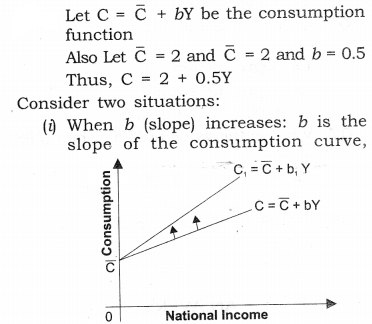when b increases from 0.5 to 0.75, then consumption curve (which is a straight line) pivots upwards. This is called a parametric shift of a graph. It is shown in figure.
(ii)When C increases: It is the autonomous part of the consumption function. It graphically gives the intercept of the consumption curve. If increases from 2 to 5, there will be parallel upward shift in the consumption curves. It is shown in figure given.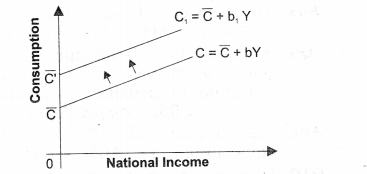Thus, when slope changes, there is parametric shift in the curve. When intercept changes, there is parallel shift in the curve.

MORE-QUESTIONS SOLVED

Question 1. What is Aggregate demand in Macroeconomics? [CBSE 2005C, 06, 09C, 10; AI 2015]
Answer: It is aggregate expenditure on ex-ante (planned) consumption and ex-ante (planned) investment that all sectors of the economy are willing to incur at each income level.

Question 2. What is Aggregate Supply in Macroeconomics?[CBSE 2015, 1995C, 2000; AI 1999]
AnswerAggregate supply is the total amount of money value of goods and services, (which is paid to the factor of production against their factor services) that all the producers are willing to supply in an economy.

Question 3. What is consumption function?[CBSE 2008]
AnswerConsumption function expresses functional relationship between aggregate consumption and national income.

Question 4. Can the value of APC be less than zero?
AnswerNo, because even at the zero level of income, we will consume something i.e., autonomous consumption.

Question 5. Why can value of MPC be not greater than one? [AI 1998]
AnswerIt is so because Keynes’ psychological law of consumption states that when income increases, consumption also increases but at a lesser rate. So, increase in consumption is always less than increase in income, i.e., MPC=ΔC/ΔY is always less than one.

Question 6. Can the value of average propensity to save be negative? If yes,when?[CBSE Foreign 2009]
AnswerYes, the value of average propensity to save can be negative when consumption is more than national income, i.e., before the break-even point.

Question 7. What can be the maximum value of marginal propensity to save?
Answer: The maximum value of marginal propensity to save is 1. It is only possible when MPC = 0, i.e., the entire additional income is saved.

Question 8. What is the relationship between APC and APS?
AnswerThe sum of APC and APS is equal to one, i.e., APC + APS = 1.

Question 9. What is the relationship between marginal propensity to save and marginal propensity to consume?[CBSE Sample Paper 2010]
AnswerThe sum total of MPC and MPS is equal to one, i.e., MPC + MPS = 1.

Question 10. Give the meaning of autonomous consumption. [CBSE 2009]
AnswerIt refers to minimum level of consumption (i.e., C) , which is needed for survival, i.e., consumption at zero level of national income.

Question 11. Let the consumption be C = 50 + 0.75 Y, then calculate saving function?
S = Y – C = Y -(50 + 0.75 Y)
– Y – 50 – 0.75Y
S = -50 + Y(l-0.75) = -50 + 0.25Y

II. Multiple Choice Questions (1 Mark)
Question 1. If APS is 0.9, how much will be the APC?
(a) 0.2 (b) 0.5
(c) 1 (d) 0.1

Question 2. If MPC is 0.6, what will be the MPS?
(a) 0.2 (b) 0.4
(c) 0.9 (d) 0.5

Question 3. If disposable income is ?1000 and consumption expenditure is ?750, the value of average propensity to
save will be——————-.
(a) 0.25 (b) 0.9
(c) 0.85 (d) 0.2

Question 4. If the saving function is S = -50 + 0.2Y, then the MPC is
(a) 0.45 (b) 0.8
(c) 0.65 (d) 0.25

Question 5. There is parametric shift in the consumption line when —————.
(a) slope changes
(b) intercept changes
(c) APC changes
(d) none of them

Question 6. Guideline is the alternative name of ——————.
(a) aggregate demand
(b) aggregate supply
(c) MPC (d) APS

Question 7. What is the value of MPC when MPS = 0?
(a) 0 (b) 4
(c) 3 (d) 1

Question 8. If MPS is one, how much is MPC?
(a) 3 (b) 2
(c) 0 (d) 1

III. Short Answer  Type Questions [3-4 Marks]
Question 1. Explain the components of aggregate demand.
Or
State components of AD. Describe any one.[CBSE 1995, 96, 96C, 97C, 2005C, 06, 09]

AnswerThe components of aggregate demand are:

1. Private (or Household) consumption demand
(a) The total expenditure incurred by all the households of the countiy on their personal consumption is known as private consumption expenditure.
(b) Consumption demand depends mainly on disposable income and propensity to consume.
2. Private investment demand
(a) Private investment demand refers to the demand for capital goods by private investors.
(b) It is addition to the existing stock of real capital assets such as machines, tools, factory-building etc.
(c) Investments demand depends upon marginal efficiency of capital (Marginal efficiency of investment) and interest rate.
(d) Investment is of two types, Autonomous Investment and Induced investment, but in Keynes theory investment assumed to be Autonomous.
3. Government demand for goods and services
(a) In a modem economy, the government is an important buyer of goods and services.
(b) The government demand may be on account of public needs for roads, schools, hospitals, power, irrigation etc, for the maintenance of law and order and for defence.
4. Demand for net export (X – M)
(a) Net export represents foreign demand for goods and services produced by an economy.
(b) When exports exceed imports, net exports is positive and when imports exceed, net exports is negative.
(c) Exports and imports of a country are influenced by a number of factors such as foreign trade policy, exchange- rate, prices and quality of goods etc.
Thus, aggregate demand consists of these four types of demand.
AD = C + I + G + (X – M)

Question 2. Explain the distinction between ‘autor mous investment’ and “induced investment’. [CBSE 2013 (C)]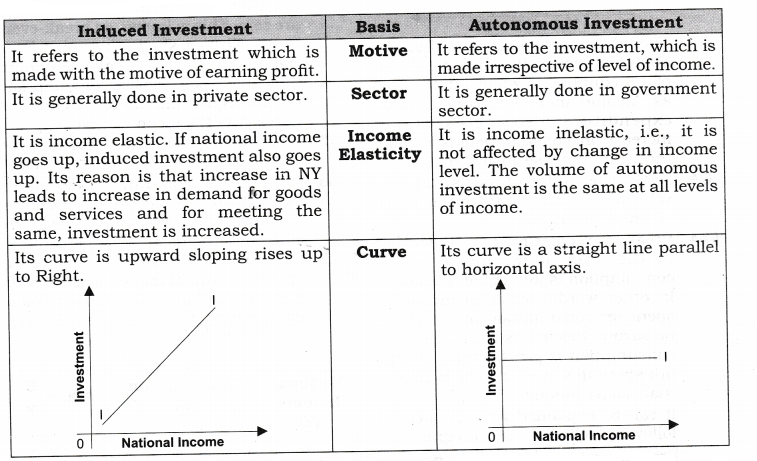Question 3. Briefly state the concept of consumption function. Explain with schedule and diagram. [CBSE 2008; AI 08, 09]
Answer:  (i) Consumption function expresses functional relationship between aggregate consumption and national income. Thus, consumption (C) is a function of income (Y).
C = F (Y)
Where,
C = Consumption
F = Function
Y = Disposable income
(ii) It can be explained with the help of the following schedule and diagram: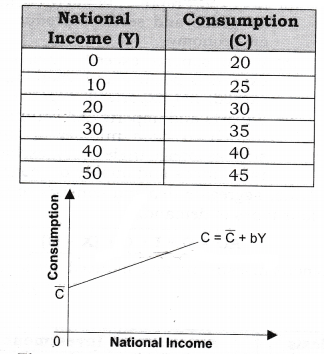The above schedule and diagram shows Keynes’ Psychological law of Consumption, which states that as income increases consumption expenditure also increases but increase in consumption is smaller than the increase in income.

Question 4. With the help of consumption schedule or curve bring out meaning of break-even point.
Answer:  Break-even point refers to that point in the level of income at which consumption is just equal to income. In other words, whole of income is spent on consumption and there is no saving. Below this level of income, consumption is greater than income but above this level, income is greater than consumption.
It can be explained with the help of following schedule and diagram: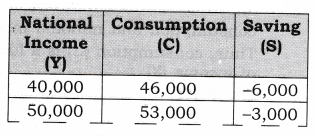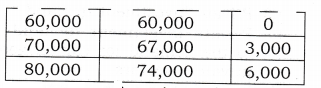In the above imaginary house hole schedule of consumption and saving, at annual income level of Rs 60,000, consumption is Rs 60,000 and in consequence there is no saving. This is break-even point.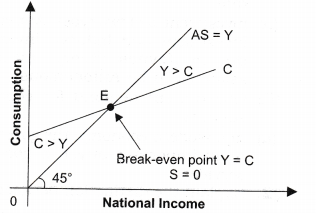In the above diagram, when Consumption (C) = National Income(Y), savings are zero. This is known as break-even point. This is shown by point E in the diagram. Thus break even point indicates a point where consumption becomes equal to income or consumption curve cuts the income curve.

Question 5. What is APC? How is it calculated?[AT 1991; CBSE 92 C, 2004]
AnswerThe ratio of aggregate consumption expenditure to aggregate income is known as average propensity to consume. It indicates the percentage (or ratio) of income which is being spent on consumption. It is worked out by dividing total consumption expenditure (C) by total income (Y). APC=C/Y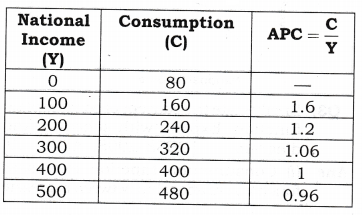Question 6. Distinguish between APS and MPS. The value of which of these two can be negative and when? [CBSE 2004, 2011]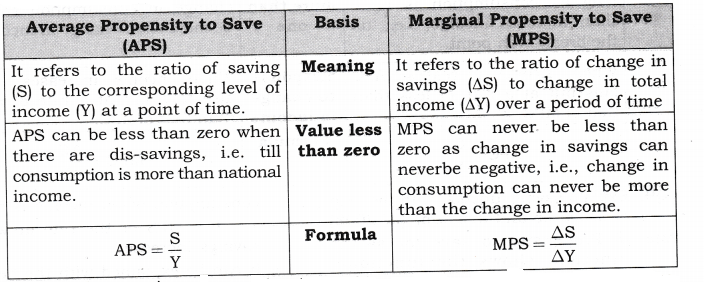APS can be negative, when at low level of income consumption exceeds income, savings are negative which make the APS negative. It can be explained with the help of the following schedule: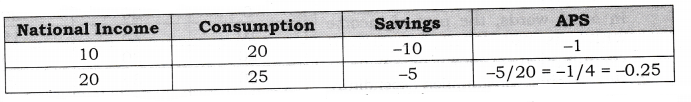Question 7. Differentiate between APC and APS and tell which of them is negative.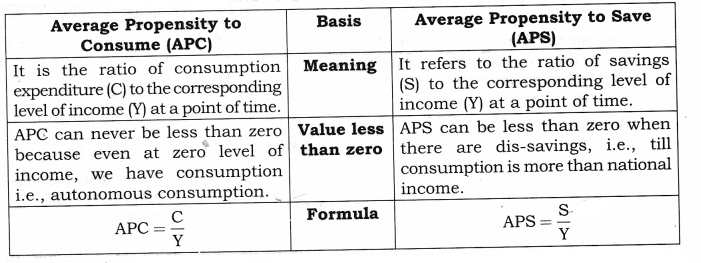APS can be negative. When at low level of income consumption exceeds income, savings are negative and make the APS negative. It can be explained with the help of the following schedule.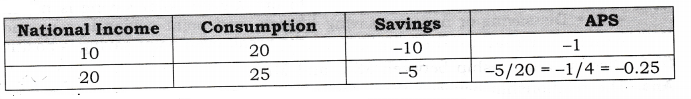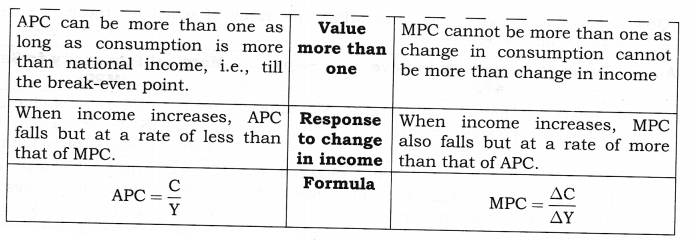Question 9. Explain saving function with the help of schedule and diagram. [AI 2008]
Answer: (i) Propensity to save (or saving function) shows the functional relationship between aggregate savings and income.S=f(Y)
In other words, the part of income which is not spent on current consumption is known as saving. By deducting consumption expenditure (C) from income (Y), we get saving (S). S = Y – C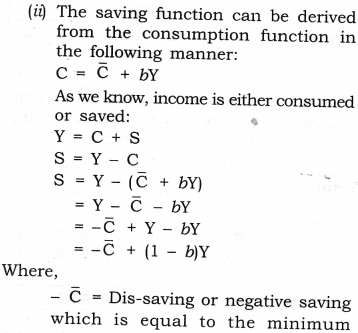IV.True Or False
Giving reasons, state whether the following statements are true or false:
Question 1. Average propensity to save is always greater than zero. [CBSE (D) 2010]
Answer: False. Because at very low level of income, when consumption exceeds income, then saving becomes negative and APS < 0. It can be explained with the help of the following schedule: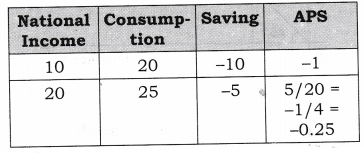Question 2. Value of average propensity to save can never be less than zero.[CBSE AT 2010]
Answer: False. The value of APS can be less than zero (i.e., negative). When consumption expenditure (C) becomes greater than income then the volume of savings becomes negative. Hence APS will be negative, (i.e., less than zero). This is shown as;
Let C = 1500 and Y = 1,000.
We know, S = Y – C = 1,000 – 1500 = -500
APS=S/Y = -500/1000 =(-)0.5
Thus, the value of APS can be less than zero (i.e. negative).

Question 3. When the value of average propensity to save is negative, the value of marginal propensity to save will also be negative. [AI 2010]
Answer: False. Value of APS has no relationship with MPS. APS is closely related with APC, i.e., APS + APC = 1. When APC is greater than unity (as in case of lower level of income), the value of APS is negative.

Question 4. The value of marginal propensity to save can never be negative.[CBSE (D) 2010]
Answer: True. MPS can never be less than zero as change in savings can never be negative, i.e., change in consumption can never be more than the change in income.

Question 5. The value of average propensity to save can never be greater than 1.[CBSE (Fj 2010]
AnswerTrue because Saving can never be greater than Income.

Question 6. Sum of average propensity to consume and marginal propensity to consume is always equal to 1.[CBSE AI 2010]
AnswerFalse. The value of APC has no relationship with MPC. Either APC + APS = 1 or MPC + MPS = 1

Question 7. If income is Rs 10,000 crore and consumption is Rs 5,000 crore, APC would be 0.5.
Note: As per CBSE guidelines, no marks will be given if reason to the answer is not explained.

V.Long Answer Type Questions (6 Marks)
Question 1. Draw a straight line consumption curve. From it derive the saving curve. Explain the process of derivation on the diagram, show:
(i) The income level at which APC =1.
(ii) The income level at which APS is negative. [CBSE Sample Paper 2014] Or
Outline the steps taken in deriving saving curve from the consumption curve. Use diagram. [CBSE 2012] Or
Draw on a diagram a straight line Consumption curve for an economy. From it derive the saving curve, explaining the method of derivation. Show a point on the consumption curve at which APS = 0?

Answer:  To explain the below figure we define the following two terms.
(i) Consumption function: Consumption function expresses functional relationship between aggregate consumption and national income. It can be expressed as:C¯ =C+bY C¯
where =C¯[latex]C¯ =Autonomous consumption, b= marginal propensity to consume.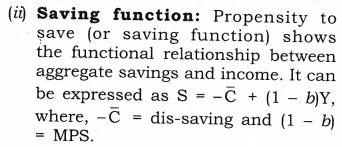(iii) Figure B is derived from figure A. In Figure A at point P1 consumption is equal to national income, which is known as break-even point. At point P, APC = 1 because consumption is equal to income at this point.
(iv) Corresponding to point P, we derive the point P1in figure B where Saving is equal to zero. At point P1APS =0. After point P in figure A, national income is greater than consumption,i.e., positive saving, which has shown in figure B, after point P1, where savings are positive.
(v) Before point P in figure A consumption is greater than income, i.e., negative saving or dis-saving, which has been shown in figure B before point P1 where savings are negative.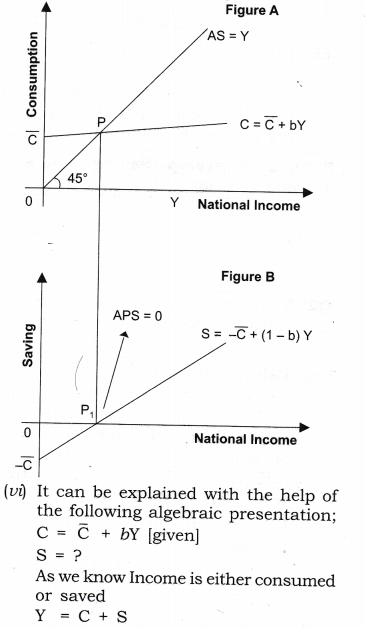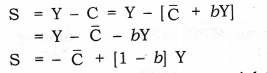Question 2. Draw on a diagram a straight line savings curve for an economy. From it derive the consumption curve, explaining the method of derivation. Show a point on the consumption curve at which APC =1? [CBSE 2006]
AnswerTo explain the above figure we define the following two terms.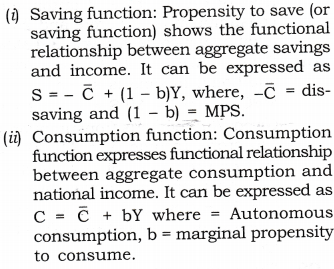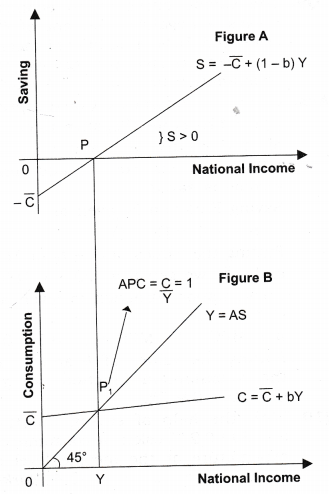(iii) Figure B is derived from A. In Figure A at point P saving = 0. Corresponding to point P, we derive the point P: in figure B where income = consumption. Point Pj is known as to be break-even point and at this point only APC = 1.
(iv) After point P in figure A savings are positive, which has been shown in figure B, after point Pt where Income is greater than consumption, i.e., positive saving.
(v)  Before point P in figure A savings are negative which has shown in figure B before point Pi; where consumption is greater than income, i.e., negative saving or dis-saving.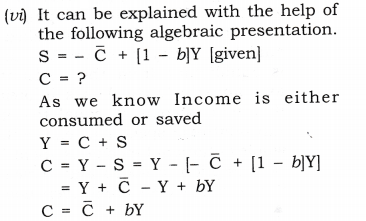VI. Higher Order Thinking Skills
Question 1. Why APC falls with the increase in income? [ 1 Mark]
Answer: APC falls continuously with the increase in income because the proportion of income spent on consumption keeps on decreasing.

Question 2. Can APC be zero? [1 Mark]
AnswerAPC can be zero only when consumption becomes zero. However, consumption is never zero at any level of income. Even at zero level of national income, there is autonomous consumption (C ).

Question 3. What can be the maximum value of marginal propensity to save? [ 1 Mark]
Answer: The maximum value of marginal propensity to save is 1. It is possible only when MPC = 0, i.e., the entire additional income is saved.

Question 4. MPC of the poor is more than that of the rich. [1 Mark]
Answer: True. It happens because the poor spend a greater percentage of their increased income on consumption as most of their basic needs remain unsatisfied. As against it, the rich people spend a smaller proportion as their basic needs are already satisfied.

Question 5. In Keynes theory, MPC fall with an increase in income. [1 Mark]
Answer: False. Because Keynes assumed MPC remains constant. So, with the increase in income, MPC remains constant.

Question 6. What is a guideline? [3 Marks]
AnswerThe aggregate supply (AS) curve which is a 45° positively sloping line from the origin. -It is also called a guideline. Every point on AS curve is equidistant from the horizontal axis and the vertical axis implying that total income is equal to total expenditure or spending, i.e., C + S. If whole of national income is spent on consumption of goods and services, S = 0. It is called break-even point.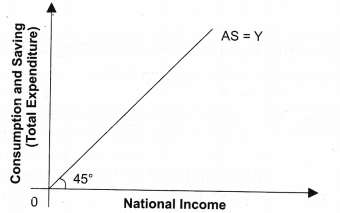VII. Value Based Question
Question 1. Why do the consumption expenditure of involuntary unemployed worker is not zero, even at zero level of income?[1 Mark]
AnswerA worker has to incur some expenditure to fulfill his basic needs even at zero level of income.
Value: Analytic.

VIII. Applications Based Questions
Question 1. Given below is the consumption function of an economy:C = 100 + 0.5Y.
With the help of a numerical example show that in this economy as income increases APC will decrease.[CBSE Sample Paper 2010][6 Marks]

Given consumption function: C = 100 + 0.5Y.
To show that APC decreases with the increase in income, consider the following hypothetical schedule: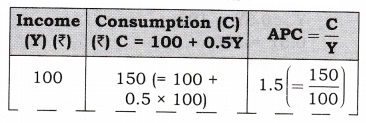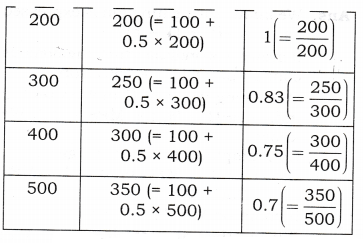As seen in the given schedule when the income increases from Rs 100 crore to Rs 200 crore and then to Rs 300 crore and so on, APC decreases from 1.5 to 1 and then to 0.83 and keeps on decreasing with the increase in income. Therefore, with an increase in income, APC decreases.

Question 2. What do you understand by ‘parametric shift of a line? How does a line shift when its (i) slope decreases and (ii) its intercept decreases? [3-4 Marks]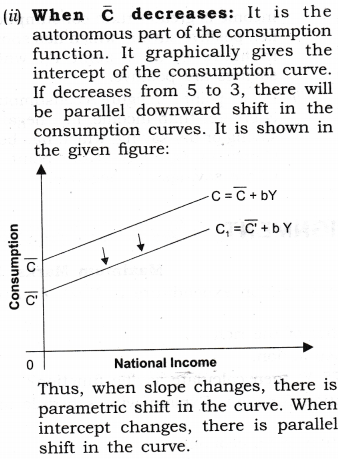Question 3. “Economists are generally concerned about the rising Marginal Propensity to Save (MPS) in an economy”. Explain why?[CBSE Sample Paper 2016] [3-4 Marks]

1. As we know, the sum of MPC and MPS is unity. Any Increase in Marginal Propensity to save (MPS) would leads to decrease in Marginal Propensity to consume (MPC).
2. It means, with the increase in Income, lesser and lesser proportion of Income is spend on consumption.
3. As, consumption is an important component of Aggregate Demand. If consumption falls, Aggregate Demand/Expenditure Fall, which will further leads to fall in Equilibrium level of Income in the economy.

Question 4. Derive a straight line saving curve using the following consumption function:
C = 200 + 0.6 Y. Presuming the income levels to be Rs 100, Rs 200 and Rs 300 crores. Also calculate that level of income where consumption is equal to income.[CBSE Sample Paper 2016] [6 Marks]